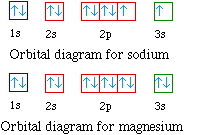### For example hydrogen with one electron has an electron configuration of 1s1. The orbital diagram for hydrogen can be represented in the following way.### This notation uses a box to represent the orbital, the label for the orbital and an arrow to represent the electron. The electronic configuration for hydrogen can be written as 1s1. This is a short-hand notation which identifies the level, the sublevel and the number of electrons in the sublevel. We can also display the energy level diagram for the hydrogen atom. A portion of the energy level diagram is shown,### The next atom is helium with 2 electrons. So the second electron could go into the 1s orbital with the opposite spin of the first electron or it could go into the next orbital in the n = 2 level. It turns out that the energy required to accommodate two electrons in the 1s orbital is significantly less than the energy required to place the second electron into the higher energy n = 2 level. The orbital diagram for helium is,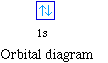### So while hydrogen has the electron configuration of 1s1, helium has the electron configuration of 1s2. The energy diagram for helium is shown as here. Notice that there has been a change in the relative energies of the 2s and 2p orbitals. This is an important point that must be addressed at this point.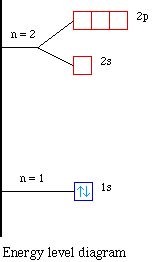### So the orbital diagram for lithium is shown below. The electron configuration for lithium is 1s22s1.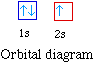### The energy level diagram, on the left shows the relative energy of the 2s and 2p orbitals based on the ability of the sublevels to penetrate to the nucleus.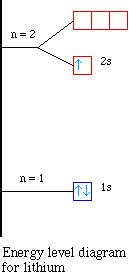### The next element is beryllium which has four electrons. The orbital diagram for beryllium is shown here. The electron configuration is 1s22s2. The fourth electron is placed in the 2s orbital. The energy required to pair the first 2s electron is less than the energy required to place the electron into the 2p orbital.### The next element is boron with 5 electrons. The orbital diagram for boron as shown has the one electron in the 2p orbital. The electron can be placed in any of the three 2p orbitals. The electron configuration for boron is 1s22s22p1.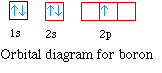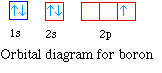### The energy level diagram for boron is show below.### The electron configuration for carbon is 1s22s22p2. Notice the electron configuration does not clearly indicate the number of unpaired electrons in the element. The number of unpaired electrons is evident from the orbital diagram. The orbital energy diagram for carbon is shown below.### Nitrogen has seven electrons. The placement of the next electron must follow Hund's rule. The orbital diagram shows three unpaired electrons. The electron configuration for nitrogen is 1s22s22p3.### For oxygen the eighth electron must pair with one of the electrons in the 2p orbitals. The orbital diagram for oxygen is shown on the left. The electron configuration for oxygen is 1s22s22p4.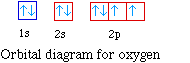### The orbital diagrams for fluorine and neon are shown. The next two electrons continue to pair those electrons that are unpaired to fill up the 2p orbitals.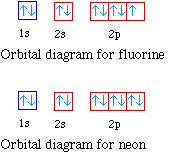### The orbital diagrams for the eight elements are shown below.